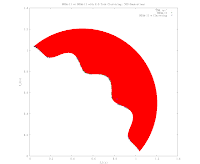## Tuesday, October 4, 2011

### The Continuing Adventures of NSGA-II

I have implemented clustering inside the official NSGA-II implementation in C. It seems to converge faster and with more spread than the baseline:The trick is processing these results to determine whether the apparent convergence is real or not. The only way I know to test the convergence and distribution is to use the Upsilon (average distance to Pareto frontier) and Delta (distances to neighbors weighted by average distances) measures Deb used, but these require knowing the mathematical definition of the Pareto frontier to calculate equally distributed points across it.

To approximate this, I tried writing an incremental search through the problem space using kd-clustering to refine the search space. To my surprise, it did not seem to work well:So, I've tried switching to use calculus to determine these points by hand. This is not going well, either:

Schaffer Pareto Frontier: f2 = (sqrt(f1) - 2)^ 2
Area under Frontier: Integral fx dx = 1/2 x^2 - 8/3 x sqrt(x) + 4x + C
Calculating x's that generate precise areas... ?